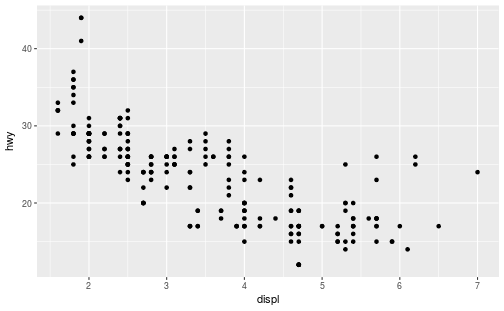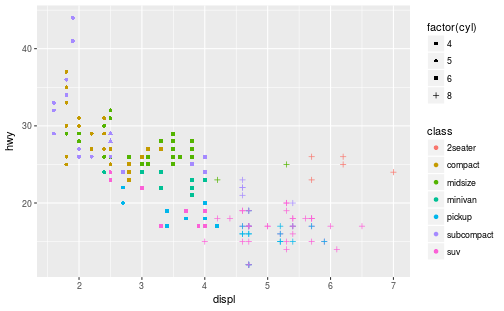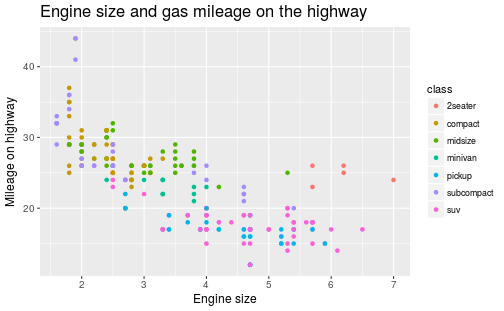# Materials for Class 5

## General

### Annoucements

• Next set of readings to be posted soon.
• Homework 1 will be posted in the next day or so.

## Last time…

### Data visualization as communication

• Effective communication ↔ effective visuals

• Difference between a clear message about your data versus a confusing one

• Important decisions can hinge on how persuaded people are with your presented work!

• Going against plotting conventions, even if the data is literally accurate, can be misleading

• Caveat emptor: breaking visual conventions can be a deliberate strategy, approach with caution and careful skepticism

### Principles and ethics for scientific visualizations

1. Present your results transparently and honestly
2. Show all data, including outliers, that are valid measurements
3. Use graph layouts that show trends and lets readers easily read quantitative values
4. Do not break conventions regarding scaling, axis orientation, the type of plot to use, etc.
5. If you leave something out of a visualization, say so and justify it
6. Strongly consider including your datasets and any scripts used to create figures with your reports or journal articles

### Data visualization as exploration

library(tidyverse)
mpg
manufacturer model displ year cyl trans drv cty hwy fl class
audi a4 1.8 1999 4 auto(l5) f 18 29 p compact
audi a4 1.8 1999 4 manual(m5) f 21 29 p compact
audi a4 2.0 2008 4 manual(m6) f 20 31 p compact
audi a4 2.0 2008 4 auto(av) f 21 30 p compact
audi a4 2.8 1999 6 auto(l5) f 16 26 p compact
audi a4 2.8 1999 6 manual(m5) f 18 26 p compact

### The scatterplot

Plot each car’s highway fuel efficiency (hwy) as a function of the engine size (displ):

ggplot(data = mpg) +
geom_point(mapping = aes(x = displ, y = hwy))### Layering details

ggplot(data = mpg) +
geom_point(mapping = aes(x = displ, y = hwy, color = class, shape = factor(cyl)))## How to describe visualizations

### A taxonomy for data graphics

We can break visualizations down into four basic elements:

• Visual cues

• Coordinate system

• Scale

• Context

### Visual cues

• These are the building blocks of any given visualization.

• Identify 9 separate visual cues.

### Cues 1–3

1. Position (numerical) where in relation to other things?

2. Length (numerical) how big (in one dimension)?

3. Angle (numerical) how wide? parallel to something else?

### Cues 4–6

1. Direction (numerical) at what slope? In a time series, going up or down?

2. Shape (categorical) belonging to which group?

3. Area (numerical) how big (in two dimensions)?

### Cues 7–9

1. Volume (numerical) how big (in three dimensions)?

2. Shade (either) to what extent? how severly?

3. Color (either) to what extent? how severly? Beware of red/green color blindness.

### Coordinate systems

1. Cartesian This is the familiar (x, y)-rectangular coordinate system with two perpendicular axes

2. Polar: The radial analog of the Cartesian system with points identified by their radius ρ and angle θ

3. Geographic: Locations on the curved surface of the Earth, but represented in a flat two-dimensional plane

### Scale

1. Numeric: A numeric quantity is most commonly set on a linear, logarithmic, or percentage scale.

2. Categorical: A categorical variable may have no ordering or it may be ordinal (position in a series).

3. Time: A numeric quantity with special properties. Because of the calendar, it can be specified using a series of units (year, month, day). It can also be considered cyclically (years reset back to January, a spring oscillating around a central position).

### Context

Annotations and labels that draw attention to specific parts of a visualization.

• Titles, subtitles

• Axes labels that depict scale (tick mark labels) and indiciate the variable

• Reference points or lines

• Other markups such as arrows, textboxes, and so on (it’s possible to overdo these)

### Example plot

How many of the previous elements can you identify in this plot?## More on ggplot2

Split your plot into “facets”; particularly useful for categorical variables.

ggplot(data = mpg) +
geom_point(mapping = aes(x = displ, y = hwy)) +
facet_wrap( ~ class)

Also try:

facet_wrap( ~ class, nrow = 2)
facet_grid(drv ~ cyl)
facet_grid(drv ~ .)
facet_grid(. ~ cyl)

Don’t forget the + sign!

### Using geom_smooth

We use geom_smooth to dip our toe into the world of data-driven modeling.

What do you get when you run the following?

ggplot(data = mpg) +
geom_point(mapping = aes(x = displ, y = hwy)) +
geom_smooth(mapping = aes(x = displ, y = hwy))

To use the more familiar linear model (the so-called “line of best fit”), include the input method = "lm".

ggplot(data = mpg) +
geom_point(mapping = aes(x = displ, y = hwy)) +
geom_smooth(mapping = aes(x = displ, y = hwy), method = "lm")